Published

# Engine & Transmission Temperature Gauges on a Budget

Don't spend hundreds of dollars on aftermarket temperature sensors or rely on a fake gauge ever again!

BeginnerFull instructions provided2.5 hours1,646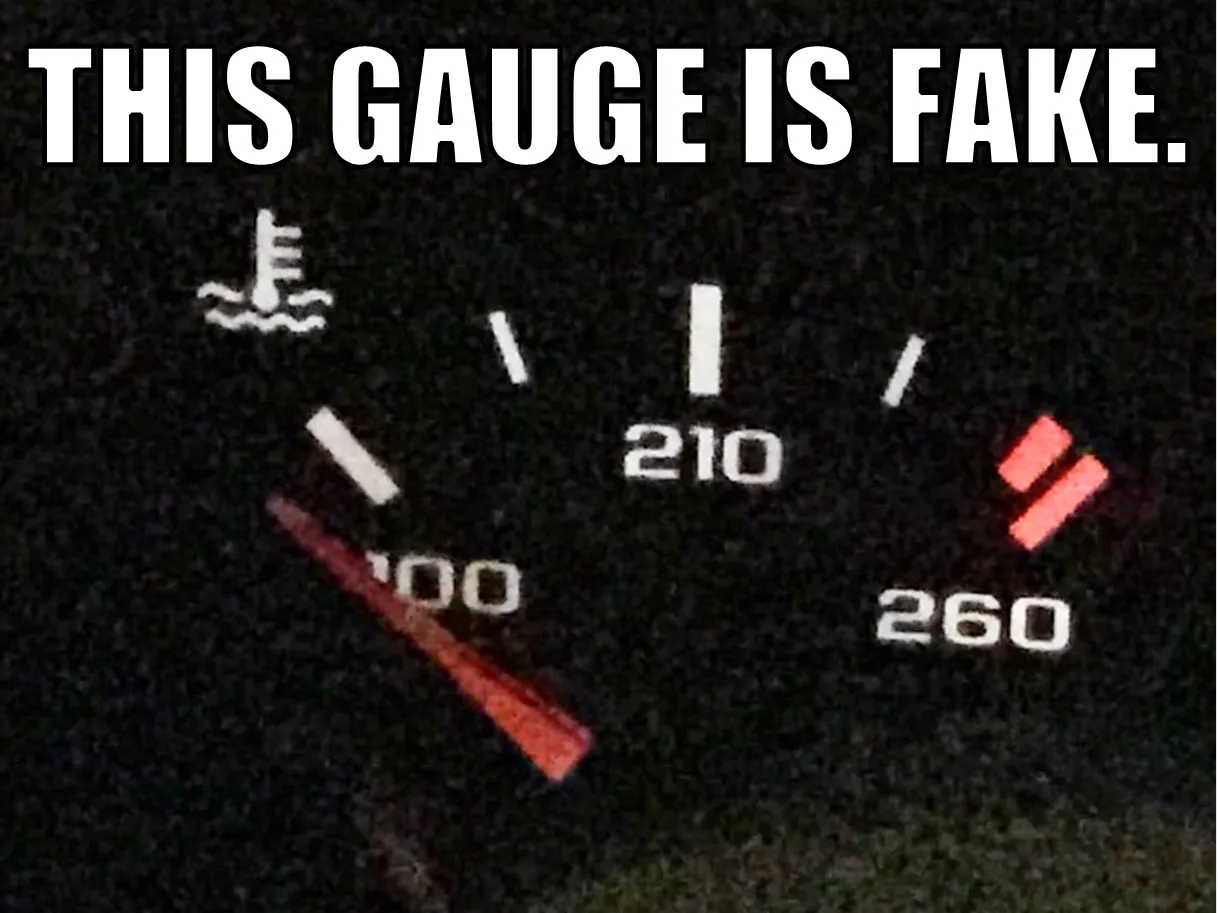## Things used in this project

### Hardware componentsParticle Photon
×2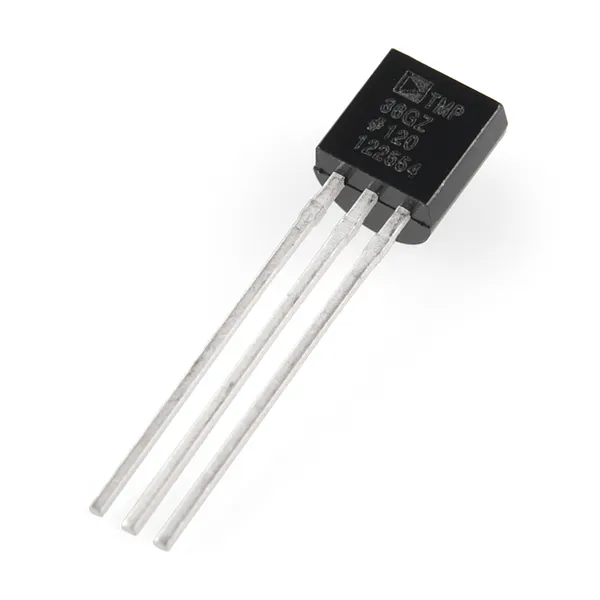Temperature Sensor Waterproof, 2m, http://a.co/jezaC9W
×2Jumper wires (generic)
×3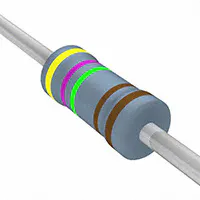Resistor 4.75k ohm
×1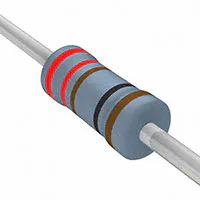Resistor 221 ohm
×1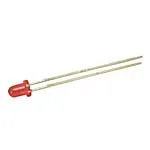3 mm LED: Red
×1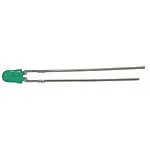3 mm LED: Green
×1×2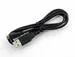USB-A to Micro-USB Cable
×2

### Hand tools and fabrication machinesSoldering iron (generic) Soldered Wires to each of the ends of the temp sensors and covered with electrical tape.
 QuikSteel Used to hold sensors to the transmission and engine oil pan.

## Schematics

### 1 - Temperature Measuring Particle

Note: Waterproof Temperature Sensors are used for this, just connect the colored wires on there where you see the colored wires on here. Also, two one-wire temperature sensors can be on the exact same lines to transmit data, which is shown here. The second sensor does not need to be all the way back as shown. The resistor used is 4.7k Ohm and is connecting the yellow and red sides together.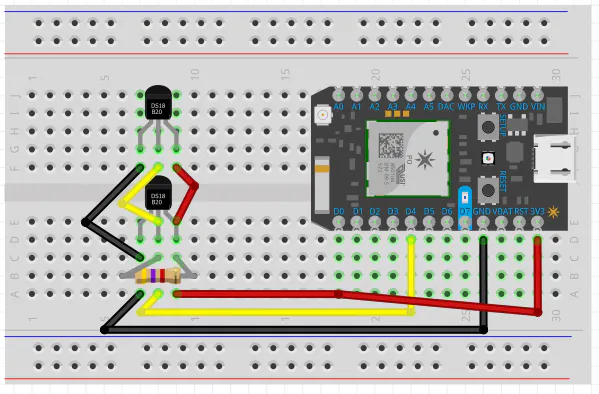### 2 - LED Particle

D7 itself is an LED. The LED's used are red and green and 3mm in size. Note that the longer leg of the LED's are the positive side and MUST be the ones to stick connect to the pins on the particle. A 220 Ohm resistor connects both negative sides to ground.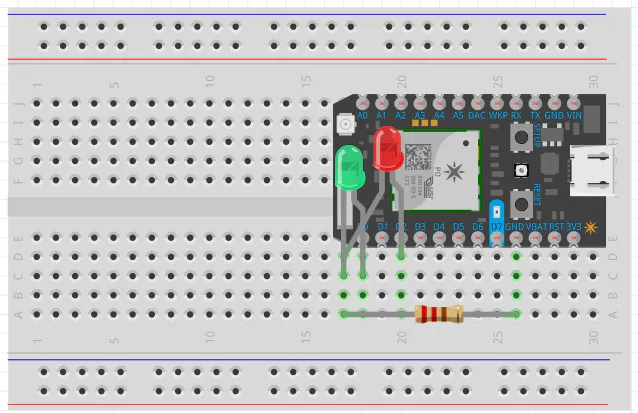## Code

### 2 - LED Display Particle

C/C++
This simple code receives the data from the first particle and will turn on/off LEDs to alert you on the car's temperature.
```void setup()
//These pinModes are for the LED's. You can change them as you wish.
{
pinMode(D0, OUTPUT);
pinMode(D7, OUTPUT);
pinMode(D2, OUTPUT);
Particle.subscribe("insert_your_event_here", myHandler);
}
void loop () {

}
//atoi converts the data back into temperature. The program works by
//taking temp measurements and comparing it to turn on or off LED's.
//Change the temp vlaues as you desire.
//We've found that both the engine and transmission have usually warmed up
//By the time that both have hit 110 deg. Keep in mind that the sensors are
//on the outside and both air cooling and rate of heat transfer are considered.
void myHandler(const char *event, const char *data) {
int num = atoi(data);
if(num<=110) {
digitalWrite(D7,HIGH);
digitalWrite(D0,LOW);
digitalWrite(D2,LOW);
}
else if(num>110 && num<210) {
digitalWrite(D2,LOW);
digitalWrite(D0,HIGH);
digitalWrite(D7,LOW);
}
else {
digitalWrite(D7,LOW);
digitalWrite(D0,LOW);
digitalWrite(D2,HIGH);
}
}
```

### 1 - Temperature Measuring/Publishing Particle

C/C++
This code is nearly the exact same as the Particle Tutorial Code on Temperature Logging. Be sure to add the OneWire App and adjust the publishing settings at the very bottom in order for it to work.
```//Go to Libaries, OneWire, and DS18x20_Temperature.ino.
//Then Click add to app to add it to this code FIRST.
//Otherwise, this code is nearly identical to the code
//found on the Particle Tutorial for temperature Logging.
#include "OneWire/OneWire.h"

OneWire ds = OneWire(D4);

unsigned long lastUpdate = 0;

void setup() {
Serial.begin(9600);
pinMode(D3, OUTPUT);
pinMode(D5, OUTPUT);
digitalWrite(D3, LOW);
digitalWrite(D5, HIGH);
}

void loop(void) {
byte i;
byte present = 0;
byte type_s;
byte data;
float celsius, fahrenheit;

Serial.println();
ds.reset_search();
delay(250);
return;
}

Serial.print("ROM =");
for( i = 0; i < 8; i++) {
Serial.write(' ');
}

Serial.println("CRC is not valid!");
return;
}
Serial.println();

case 0x10:
Serial.println("  Chip = DS1820/DS18S20");
type_s = 1;
break;
case 0x28:
Serial.println("  Chip = DS18B20");
type_s = 0;
break;
case 0x22:
Serial.println("  Chip = DS1822");
type_s = 0;
break;
case 0x26:
Serial.println("  Chip = DS2438");
type_s = 2;
break;
default:
Serial.println("Unknown device type.");
return;
}

ds.reset();
ds.write(0x44, 0);

delay(1000);

present = ds.reset();
ds.write(0xB8,0);
ds.write(0x00,0);

present = ds.reset();
ds.write(0xBE,0);
if (type_s == 2) {
ds.write(0x00,0);
}

Serial.print("  Data = ");
Serial.print(present, HEX);
Serial.print(" ");
for ( i = 0; i < 9; i++) {
Serial.print(data[i], HEX);
Serial.print(" ");
}
Serial.print(" CRC=");
Serial.print(OneWire::crc8(data, 8), HEX);
Serial.println();

int16_t raw = (data << 8) | data;
if (type_s == 2) raw = (data << 8) | data;
byte cfg = (data & 0x60);

switch (type_s) {
case 1:
raw = raw << 3;
if (data == 0x10) {
raw = (raw & 0xFFF0) + 12 - data;
}
celsius = (float)raw * 0.0625;
break;
case 0:
if (cfg == 0x00) raw = raw & ~7;
if (cfg == 0x20) raw = raw & ~3;
if (cfg == 0x40) raw = raw & ~1;
celsius = (float)raw * 0.0625;
break;

case 2:
data = (data >> 3) & 0x1f;
if (data > 127) {
celsius = (float)data - ((float)data * .03125);
}else{
celsius = (float)data + ((float)data * .03125);
}
}

fahrenheit = celsius * 1.8 + 32.0;
Serial.print("  Temperature = ");
Serial.print(celsius);
Serial.print(" Celsius, ");
Serial.print(fahrenheit);
Serial.println(" Fahrenheit");

//This is really all you need to play around with here. Just name it what you want and change the delay (in miliseconds)
//for recording. Remember only one sensor is read per delay cycle, so take that into account.
String temperature = String(fahrenheit);
Particle.publish("insert_your_event_here", temperature, PUBLIC);
delay(15000);
}
```

## Credits

Thanks to Particle and Ric DelMar.